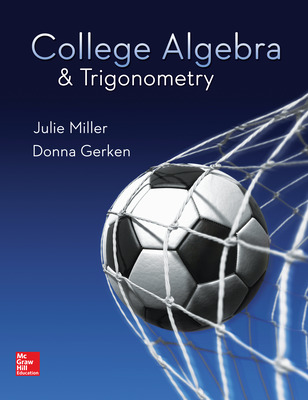# College Algebra & Trigonometry

1st Edition
By Julie Miller and Donna Gerken
ISBN10: 0078035627
ISBN13: 9780078035623
Product Details +

## Purchase Options

Students, we’re committed to providing you with high-value course solutions backed by great service and a team that cares about your success. See tabs below to explore options and pricing. Don't forget, we accept financial aid and scholarship funds in the form of credit or debit cards.

### McGraw-Hill eBook

Available for purchase 12/01/2020

• Rent or purchase for a fraction of the printed textbook price
• Easily highlight, take notes and search
• Note: the eBook does not include access to Connect. If your instructor assigned Connect, click the "Digital" tab.

### Hardcopy

• Bound book containing the complete text
• Full color
• Hardcover or softcover

### Hardcopy

• Bound book containing the complete text
• Full color
• Hardcover or softcover

### Hardcopy

• Bound book containing the complete text
• Full color
• Hardcover or softcover

The estimated amount of time this product will be on the market is based on a number of factors, including faculty input to instructional design and the prior revision cycle and updates to academic research-which typically results in a revision cycle ranging from every two to four years for this product. Pricing subject to change at any time.

# College Algebra & Trigonometry © 2017

### Chapter R: Review of Prerequisites

Section R.1 Sets and the Real Number Line Section R.2 Integer Exponents and Scientific Notation Section R.3 Rational Exponents and Radicals Section R.4 Polynomials and Multiplication of Radicals Problem Recognition Exercises Simplifying Algebraic Expressions Section R.5 Factoring Section R.6 Rational Expressions and More Operations on Radicals Algebra for Calculus

### Chapter 1: Equations and Inequalities

Section 1.1 Linear Equations and Rational Equations Section 1.2 Applications with Linear and Rational Equations Section 1.3 Complex Numbers Section 1.4 Quadratic Equations Problem Recognition Exercises Simplifying Expressions Versus Solving Equations Section 1.5 Applications of Quadratic Equations Section 1.6 More Equations and Applications Section 1.7 Linear, Compound, and Absolute Value Inequalities Problem Recognition Exercises Recognizing and Solving Equations and Inequalities Equations for Calculus

### Chapter 2: Functions and Relations

Section 2.1 The Rectangular Coordinate System and Graphing Utilities Section 2.2 Circles Section 2.3 Functions and Relations Section 2.4 Linear Equations in Two Variables and Linear Functions Section 2.5 Applications of Linear Equations and Modeling Problem Recognition Exercises Comparing Graphs of Equations Section 2.6 Transformations of Graphs Section 2.7 Analyzing Graphs of Functions and Piecewise-Defined Functions Section 2.8 Algebra of Functions and Function Composition

### Chapter 3: Polynomial and Rational Functions

Section 3.1 Quadratic Functions and Applications Section 3.2 Introduction to Polynomial Functions Section 3.3 Division of Polynomials and the Remainder and Factor Theorems Section 3.4 Zeros of Polynomials Section 3.5 Rational Functions Problem Recognition Exercises Polynomial and Rational Functions Section 3.6 Polynomial and Rational Inequalities Problem Recognition Exercises Solving Equations and Inequalities Section 3.7 Variation

### Chapter 4: Exponential and Logarithmic Functions

Section 4.1 Inverse Functions Section 4.2 Exponential Functions Section 4.3 Logarithmic Functions Problem Recognition Exercises Analyzing Functions Section 4.4 Properties of Logarithms Section 4.5 Exponential and Logarithmic Equations Section 4.6 Modeling with Exponential and Logarithmic Functions

### Chapter 5: Trigonometric Functions

Section 5.1 Angles and Their Measure Section 5.2 Right Triangle Trigonometry Section 5.3 Trigonometric Functions of Any Angle Section 5.4 Trigonometric Functions Defined on the Unit Circle Section 5.5 Graphs of Sine and Cosine Functions Section 5.6 Graphs of Other Trigonometric Functions Problem Recognition Exercises Comparing Graphical Characteristics of Trigonometric Functions Section 5.7 Inverse Trigonometric Functions

### Chapter 6: Analytic Trigonometry

Section 6.1 Fundamental Trigonometric Identities Section 6.2 Sum and Difference Formulas Section 6.3 Double-Angle and Half-Angle Formulas Section 6.4 Product-to-Sum and Sum-to-Product Formulas Section 6.5 Trigonometric Equations

### Chapter 7: Applications of Trigonometric Functions

Section 7.1 Applications of Right Triangles Section 7.2 The Law of Sines Section 7.3 The Law of Cosines Problem Recognition Exercises Solving Triangles Using a Variety of Tools Section 7.4 Harmonic Motion and Combinations of Trigonometric Functions

### Chapter 8: Trigonometry Applied to Rectangular and Polar Coordinate Systems and Vectors

Section 8.1 Polar Coordinates Section 8.2 Graphs of Polar Equations Problem Recognition Exercises Comparing Equations in Polar and Rectangular Form Section 8.3 Complex Numbers in Polar Form Section 8.4 Vectors Section 8.5 Dot Product

### Chapter 9: Systems of Equations and Inequalities

Section 9.1 Systems of Linear Equations in Two Variables and Applications Section 9.2 Systems of Linear Equations in Three Variables and Applications Section 9.3 Partial Fraction Decomposition Section 9.4 Systems of Nonlinear Equations in Two Variables Section 9.5 Inequalities and Systems of Inequalities in Two Variables Problem Recognition Exercises Equations and Inequalities in Two Variables Section 9.6 Linear Programming

### Chapter 10: Matrices and Determinants and Applications

Section 10.1 Solving Systems of Linear Equations Using Matrices Section 10.2 Inconsistent Systems and Dependent Equations Section 10.3 Operations on Matrices Section 10.4 Inverse Matrices and Matrix Equations Section 10.5 Determinants and Cramer's Rule Problem Recognition Exercises Using Multiple Methods to Solve Systems of Linear Equations

### Chapter 11: Analytic Geometry

Section 11.1 The Ellipse Section 11.2 The Hyperbola Section 11.3 The Parabola Problem Recognition Exercises Comparing Equations of Conic Sections and General Equation Section 11.4 Rotation of Axes Section 11.5 Polar Equations of Conics Section 11.6 Plane Curves and Parametric Equations

### Chapter 12: Sequences, Series, Induction, and Probability

Section 12.1 Sequences and Series Section 12.2 Arithmetic Sequences and Series Section 12.3 Geometric Sequences and Series Problem Recognition Exercises Comparing Arithmetic and Geometric Sequences and Series Section 12.4 Mathematical Induction Section 12.5 The Binomial Theorem Section 12.6 Principles of Counting Section 12.7 Introduction to Probability